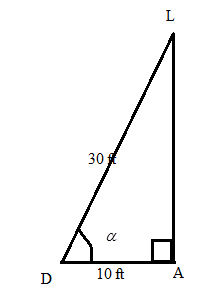Chapter 11.2, Problem 34EElementary Geometry For College St...

7th Edition
Alexander + 2 others
ISBN: 9781337614085

Solutions

Chapter
SectionElementary Geometry For College St...

7th Edition
Alexander + 2 others
ISBN: 9781337614085
Textbook Problem

In Exercise 29 to 37, angle measures should be given to the nearest degree; distances should be given to the nearest tenth of a unit.Dale looks up to see his friend Lisa waving from her apartment window 30 ft. from him. If Dale is standing 10 ft. from the building. What is the angle of elevation as Dale looks up at Lisa?.To determine

To find:

The angle of elevation as Dale looks up at Lisa by using the following condition,

“Dale looks up to see his friend Lisa waving from her apartment window 30 ft. from him. If Dale is standing 10 ft. from the building”

Explanation

Consider the following figure,

General formula for cosine ratio and sin ratio is given below,

From the given figure the value 30 ft

Still sussing out bartleby?

Check out a sample textbook solution.

See a sample solution

The Solution to Your Study Problems

Bartleby provides explanations to thousands of textbook problems written by our experts, many with advanced degrees!

Get Started

In Exercises 2730, determine all values of x for which each function is discontinuous. 30. f(x) = |2x|x

Applied Calculus for the Managerial, Life, and Social Sciences: A Brief Approach

Convert the expressions in Exercises 6584 to power form. 23(x2+1)33(x2+1)734

Finite Mathematics and Applied Calculus (MindTap Course List)

Evaluate tanxdx.

Calculus (MindTap Course List)

Evaluate the indefinite integral. cotxdx

Single Variable Calculus: Early Transcendentals, Volume I

True or False: x2 + 6x + 2y = 1 defines y as a function of x.

Study Guide for Stewart's Single Variable Calculus: Early Transcendentals, 8th